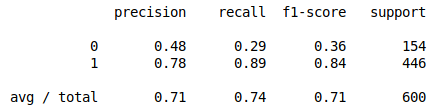# K Nearest Neighbor Classification with Python

K Nearest Neighbor uses the idea of proximity to predict class. What this means is that with KNN Python will look at K neighbors to determine what the unknown examples class should be. It is your job to determine the K or number of neighbors that should be used to determine the unlabeled examples class.KNN is great for a small dataset. However, it normally does not scale well when the dataset gets larger and larger. As such, unless you have an exceptionally powerful computer KNN is probably not going to do well in a Big Data context.

In this post, we will go through an example of the use of KNN with the turnout dataset from the pydataset module. We want to predict whether someone voted or not based on the independent variables. Below is some initial code.

```from pydataset import data
import pandas as pd
from sklearn.model_selection import train_test_split
from sklearn.neighbors import KNeighborsClassifier
from sklearn.metrics import classification_report```

We now need to load the data and separate the independent variables from the dependent variable by making two datasets.

```df=data("turnout")
X=df[['age','educate','income']]
y=df['vote']```

Next, we will make our train and test sets with a 70/30 split. The random.state is set to 0. This argument allows us to reproduce our model if we want. After this, we will run our model. We will set the K to 7 for our model  and run the model. This means that Python will look at the 7 closes examples to predict the value of an unknown example. below is the code

```X_train,X_test,y_train,y_test=train_test_split(X,y,test_size=.3,random_state=0)
clf=KNeighborsClassifier(7)
clf.fit(X_train,y_train)```

We can now predict with our model and see the results with the classification reports function.

```y_pred=clf.predict(X_test)
print(classification_report(y_test,y_pred))```The results are shown above. To determine the quality of the model relies more on domain knowledge. What we can say for now is that the model is better at classifying people who vote rather than people who do not vote.

Conclusion

This post shows you how to work with Python when using KNN. This algorithm is useful in using neighboring examples tot predict the class of an unknown example.